• C语言程序设计第八章：指针.ppt指针的概念,内存,5,int i,2000,地址变量,数据,2000,int p,指针,指针p 指向变量 i,4000,指针变量的定义和初始化,定义指针变量的一般形式如下 数据类型 *指针变量名1，*指针变量名2，；...C语言程序设计第八章：指针.ppt指针的概念,内存,5,int i,2000,地址变量,数据,2000,int p,指针,指针p 指向变量 i,4000,指针变量的定义和初始化,定义指针变量的一般形式如下 数据类型 *指针变量名1，*指针变量名2，；该指针变量可以指向何种数据类型的变量,说明符，说明其后是指针变量名例如 int *p; 定义指向整型变量的指针变量p。 float *fp; 定义指向单精度型变量的指针变量fp。 char *cp1,*cp2; 定义cp1、cp2是指向字符型变量的指针。 double *q; 定义q是指向双精度型变量的指针。,标识符满足命名规则,指针变量的赋值,(1)初始化指针变量 例如 int a; int *p 使用赋值语句为指针赋值时，前面不能再加“*”说明符，如*p p,指针的引用include void main int a,b,*p,*q; a11; b22; p,【例8-1】通过指针操作来输出变量的值和地址,指针的引用,printf“输出x和y的地址ax,bxnn“,p,q; pq; printf“将指针q的值赋给指针p后n“; printf“输出x和y的值ad,bdn“,*p,*q; printf“输出x和y的地址ax,bxnn“,p,q; ,图8-1 例8-1运行结果,【注意】 (1)*p*q;是将q指向的变量的值赋给p指向的变量，即 *p*qaba22， 此时，p和q的值都是22，p和q本身的值并未改变，p仍指向a，q指向b。 (2)pq;是将q的值赋给p，即 pqp后 (3)执行pq;后,指针的引用,表8-1 “ i5; p ,【例8-2】通过“*”与“ int *p,变量、指针变量和指向指针的指针之间的关系如图所示,指向指针的指针include include void main char c7“string“; char *p,q; forint i0;istrlenc;i p ,【例8-3】使用指向指针的指针实现程序,图8-6 例8-3运行结果,另外两种方法 include include void main char c7“string“; char *p; forint i0;i include void main char c7“string“; forint i0;istrlenc;i printf“c的地址xn“,ci, ,总结,指针是一个变量，它存储另一个对象的内存地址 指针的声明由基本类型、星号 * 和变量名组成 为指针赋值，赋值运算符右侧必须是一个地址。如果是普通变量需要在前面加一个取地址运算符 ；如果是另一个指针变量或者是一个数组，不需要加 运算符 运算符“ *” 用于返回指针指向的内存地址中存储的值 指针的算术运算的含义是指针的移动，将指针执行加上或者减去一个整数值n的运算相当于指针向前或向后移动n个数据单元 指针可以执行比较相等的运算，用来判断两个指针是否指向同一个变量 指向数组的指针，存储的是数组中元素的地址。,
展开全文• 本资源为C语言程序设计第八章课后习题加例题源代码，仅供学习分享，资源包含课后习题源代码与解题详细步骤。当然其中代码并不一定就是最完美的，因为这是作者个人做的。未经作者允许禁止分享传递。
• C程序设计中使用指针可以: 使程序简洁紧凑高效 有效地表示复杂的数据结构 动态分配内存 得到多于一个的函数返回值 & 与 * 运算符 直接访问按变量地址存取变量值 间接访问通过存放变量地址的变量去访问变量 指针变量...
• 指针的概念 内存 5 int i 2000 地址 变量 数据 2000 int p 指针 指针p 指向变量 i 4000 指针变量的定义和初始化 定义指针变量的一般形式如下 数据类型 *指针变量名1*指针变量名2 该指针变量可以指向何种数据类型的...
• 一、选择题1、对宏命令的处理是_ ___A、在程序执行时进行的B、在对程序中其他语句进行编译前进行的C、在程序连接时进行的D、与程序中其他语句同时进行编译2、下面对编译预处理的叙述正确的是_ _____A、预处理命令...
一、选择题1、对宏命令的处理是_ ___A、在程序执行时进行的B、在对程序中其他语句进行编译前进行的C、在程序连接时进行的D、与程序中其他语句同时进行编译2、下面对编译预处理的叙述正确的是_ _____A、预处理命令只能位于程序的开始处B、预处理功能是指完成宏替换和文件包含的调用C、只要行首以“#”标识的控制行都是预处理命令D、编译预处理就是对源程序进行初步的语法检查3、以下叙述中不正确的是()。A)预处理命令行都必须以#号开始B)在程序中凡是以#号开始的语句行都是预处理命令行C)C程序在执行过程中对预处理命令行进行处理D)以下是正确的宏定义#define IBM-PC4、以下叙述中正确的是()。A)在程序的一行上可以出项多个有效的预处理命令行B)使用带参的宏时,参数的类型应与宏定义时的一致C)宏替换不占用运行时间,只占编译时间D)在以下定义中C R是称为”宏名”的标识符#define C R 0455、#define ADD(x) x+xmain(){int m=1,n=2,k=3;int sum=ADD(m+n)*k;printf(“sum=%d”,sum);-上面程序的运行结果是()。A)sum=9 B)sum=10 C)sum=12 D)sum=186、以下程序的运行结果是()。#define MIN(x,y) (x)main(){int i=10,j+15,k;k+10*MIN(i,j);printf(“%d\n”,k);-A)10 B)15 C)100 D)1507、在宏定义#define PI 3.14159中,用宏名PI代替一个()。A)常量B)单精度数C)双精度数D)字符串8、以下程序的运行结果是()#include”stdio.h”#define FUDGE(y) 2.84+y#define PR(a) printf(“%d”,(int)(a))#define PRINT1(a) PR(a);put char(‘\n’)main(){int x=2;
展开全文• #include&lt;stdio.h&gt; void DivArray(int *pArray, int n); int main() { int a[] = {2,4,6,8,10,12,14},i; DivArray(a, 7); for (i = 0; i &lt; 7; i++) //问题 { printf("...void...
#include<stdio.h>
void DivArray(int *pArray, int n);
int main()
{
int a[] = {2,4,6,8,10,12,14},i;
DivArray(a, 7);
for (i = 0; i < 7; i++) //问题
{
printf("%d ",a[i]);
}
return 0;
}
void DivArray(int *pArray,int n)
{
int i;
for (i = 1; i < n; i++)//问题
{
pArray[i] /= pArray;
}
pArray /= pArray;//问题
}



展开全文• 第八章字符串 8.1字符串的定义与初始化 1.用字符数组初始化 //1.用字符数组初始化 int main() { char ch; char ch2= {'H','e','l','l','o',',','W','o','r','l','d','!'}; //如果元素的个数小于字符串...
第八章字符串
8.1字符串的定义与初始化
1.用字符数组初始化
//1.用字符数组初始化
int main()
{
char ch;
char ch2=

{'H','e','l','l','o',',','W','o','r','l','d','!'};
//如果元素的个数小于字符串长度后面会自动初始化

空字符'\0'
char ch3={'H','e','l','l','o'};
//同样可以缺省数组长度
char ch4[]=

{'H','e','l','l','o',',','W','o','r','l','d','!'};
ch='H';
ch='e';
ch='l';
ch='l';
ch='o';
ch=',';
ch='W';
ch='o';
ch='r';
ch='l';
ch='d';
ch='!';

printf("ch:%s\nch2:%s\nch3:%s\nch4:%s

\n",ch,ch2,ch3,ch4);
}

//令人不解的是ch4为什么会多出一个hello来

运行结果：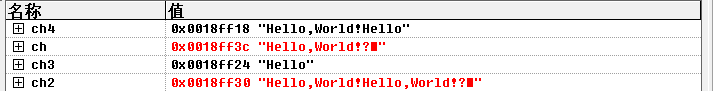2.用字符串常量初始化
#include<stdio.h>
void main()
{
char ch1={"Programing!"};
char ch2="Programing!";
char ch3[]={"Programing!"};
char ch4[]="Programing!";

printf("ch1:%s\nch2:%s\nch3:%s\nch4:%s

\n",ch1,ch2,ch3,ch4);
}

运行结果：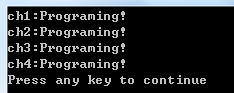3.字符数组和字符串的区别
两者不是同一个概念，只是在C语言中，用字符数组来处理字符串
void main()
{
int i;
char ch={'H','e','l','l','o',',','W','o','r','l','d','!'};
//这样打印会出错
printf("ch:%s\n",ch);
for(i=0;i<12;i++)
printf("%c",ch[i]);
printf("\n");
}

运行结果：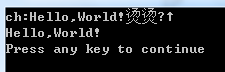8.2字符串的常用操作
8.2.1字符串的输入输出
方法1：用%c逐字输入/输出
void main()
{
int i;
char ch;
for(i=0;i<12;i++)
scanf("%c",&ch[i]);
for(i=0;i<12;i++)
printf("%c",ch[i]);
printf("\n");
}

输入Hello，World！
运行结果：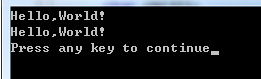方法2：用%s整体输入/输出
void main()
{
char str_a,str_b;
scanf("%s%s",str_a,str_b);
printf("%s\t%s \n",str_a,str_b);
}

输入 How are you运行结果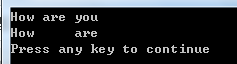提示：用%s的形式输入字符串时，字符串中不能出现空格，回车符和制表符，因为它们都是scanf默认的输入分隔符

3.用系统提供的gets和puts函数
void main()
{
char str_a;
gets(str_a);
puts(str_a);
}

输入 How are you运行结果：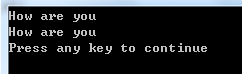8.2.2指向字符串的指针
/根据字符串的首地址来引用一个字符串，这样的指针称为字符串指针
void main()
{
char *ps;
ps="Programing!";
puts(ps);
ps="Hello,World!";
puts(ps);
}

运行结果：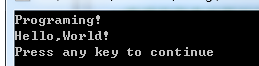注意：ch1是指针常量，不可以这样赋值
char ch1={“Hello World!”};
ch1=Programing!";

Example8.2
运用字符串指针遍历字符串，统计字母、空格、数字出现次数
void main()
{
int character=0,digit=0,space=0,other=0;
char *p="Hello!",s;
puts(p);
p=s;
printf("input string:\n");
gets(p);
while(*p!='\0')
{
if(('A'<=*p && *p<='Z') ||('a'<=*p && *p<='z'))
++character;
else if(*p==' ')
++space;
else if((*p<='9')&&(*p>='0'))
++digit;
else ++other;
p++;
}
printf("character:%d\nspace:%d\ndigit:%d\nother:%d\n",character,space,digit,other);
}


运行结果：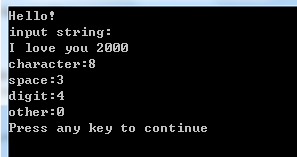Example8.3 利用二维数组读入输出多个字符串
void main()
{
char a;
int i;
for(i=0;i<5;i++)
gets(a[i]);
for(i=0;i<5;i++)
puts(a[i]);
return 0;
}

输入File<回车>Edit<回车>View<回车>Run<回车>Tools<回车>运行结果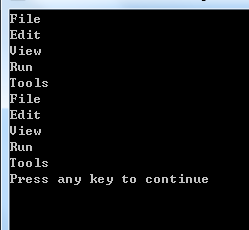8.2.3字符串处理的常用函数
unsigned int strlen(const char *str);
char *strcpy(char *destination,const char *source);
char *strcat(char *destination,const char *source);
int strcmp(const char *str1,const char *str2);
char *strupr(char *str);
char *strlwr(char *str);

8.3应用举例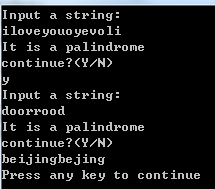8.3.1回文判断
#include<stdio.h>
#include<string.h>
#define MAX 80

int Palindrome(const char *str);
void main()
{
char str[MAX],ch;
do
{
printf("Input a string:\n");
gets(str);
if(Palindrome(str))
printf("It is a palindrome\n");
else
printf("It is not a palidrome\n");
printf("continue?(Y/N)\n");
ch=getchar();
getchar();
}while(ch=='y'||ch=='Y');
}

int Palindrome(const char *str)
{
int i=0,j=strlen(str)-1;
while(i<j)
{
while(str[i]==32)
i++;
while(str[j]==32)
j--;
if(str[j]==str[i])
{
i++;j--;
}
else return 0;
}
return 1;
}8.3.2统计单词出现次数
#include<stdio.h>
#include<string.h>

int search(const char *ps,const char *pf)
{
int count=0,i=0;
char dest;
while(*ps)
{
i=0;
while((*ps>='a' && *ps<='z') || (*ps>='A' && *ps<='Z'))
{
dest[i++]=*ps++;
}
dest[i]='\0';
ps++;
if(strcmp(dest,pf)==0)
count++;
}
return count;
}

void main()
{
char source;
char key;
puts("Input the source sentence:");
gets(source);
puts("Input the key word:");
gets(key);
printf("There are %d key words in the sentence.\n",search(source,key));
}

运行结果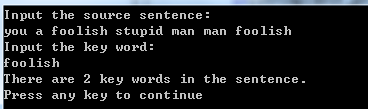8.3.3密码问题
#include<stdio.h>
#include<string.h>

int check(char *ps)
{
char passwd[]="LOVEYOU";     //设定密码
int i=0;
int flag=1;
for(;*ps!='\0' && flag;ps++)
{
if(*ps>'a' && *ps<'z')
*ps=*ps-32+2;      //解密规则
if(*ps!=passwd[i])
flag=0;
else
i++;
}
return flag;
}

void main()
{
char str;
int i=0;
printf("input your password:\n");
while((str[i]=getchar())!='#')
{
i++;
}
str[i]='\0';
if(check(str))
printf("Pass!\n");
else
printf("Error!\n \a \a \a");
}


运行结果：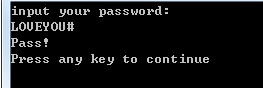8.3.4字符串的排序
方法一：用一维数组实现
#include<stdio.h>
#include<string.h>

void sort(char *str[],int n)
{
char *temp;
int i,j,k;
for(i=0;i<n-1;i++)
{
k=i;
for(j=i+1;j<n;j++)
if(strcmp(str[k],str[j])>0)
k=j;
if(k!=i)
{
temp=str[i];
str[i]=str[k];
str[k]=temp;
}
}
}

void main()
{
char *pstring={"FORTRAN","PASCAL","BASIC","C"};
int i,nNum=4;
sort(pstring,nNum);
for(i=0;i<nNum;i++)
printf("%s\n",pstring[i]);
return 0;
}


运行结果：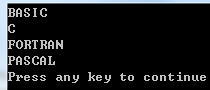方法二：用二维数组实现
#include<stdio.h>
#include<string.h>

void sort(char (*str),int n)
{
char temp;
int i,j,k;
for(i=0;i<n-1;i++)
{
k=i;
for(j=i+1;j<n;j++)
if(strcmp(str[k],str[j])>0)
k=j;
if(k!=i)
{
strcpy(temp,str[i]);
strcpy(str[i],str[k]);
strcpy(str[k],temp);
}
}
}

void main()
{
char string[]={"FORTRAN","PASCAL","BASIC","C"};
int i,nNum=4;
sort(string,nNum);
for(i=0;i<nNum;i++)
printf("%s\n",string[i]);
}


运行结果：8.4带参数的main函数

int main(int argc,char **argv)
{
int i;
for(i=1;i<argc;i++)
printf("%8s",argv[i]);
printf("\n");
return 0;
}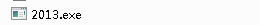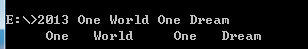展开全文• C语言会对数组下标做越界检查吗？那么我们写程序时应该注意什么？3. 对数组数据的访问一定是跟循环结构分不开的，一般情况下，循环变量的值随数组元素的下标变化。学会灵活运行循环结构完成对数组数据的处理。4.通过...
• 8.1 指针变量 8.1.1 变量的指针和指针变量 ...在C语言程序中定义一个变量，系统根据变量的类型为变量分配一定长度的存储单元。 一个存储单元手字节的编号称为该存储单元的地址，也就是相应变量的地址，在一个地
• 1、8 指针【练习8-1】如果有定义”int m, n = 5, *p = &m;”与m = n等价的语句是 B 。A m = *p; B. *p = *&n; C. m = &n; D. m = *p;解答： A:p是指向m的指针变量，所以*p等价于m。即m=m。B:&n...
• ** 利用矩阵相乘公式编程计算mn阶矩阵A和nm阶矩阵B之积 ** #include&lt;stdio.h&gt; #define ROW 2 #define COL 3 /*函数功能：计算矩阵相乘之积，结果存于二维数组c中*/ void MultiplyMatrix(int a[ROW]...
• 6.8 main函数的参数C程序的特点就是所有的程序都是用函数来装配的。main( )称之为主函数，是所有程序运行的入口。其余函数分为有参或无参两种，均由main( )函数或其它一般函数调用，若调用的是有参函数，则参数在...
• C语言程序设计(第3版)何钦铭颜晖8指针》由会员分享，可在线阅读，更多相关《C语言程序设计(第3版)何钦铭颜晖8指针(16页珍藏版)》请在人人文库网上搜索。1、8指针【练习8-1 】如果有定义” int m, n = ...
• C语言程序设计(谭浩强编)第八章 函数6学时new第8章函数 8.1 概述 函数:英文名为Function，直译为“功能”，“函数”的意思在C语言里，函数指的是实现一个特定功能的程序模块。它相当于其他语言中的子程序。 一个......

# c语言程序设计第八章c语言 订阅